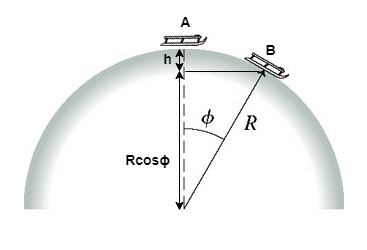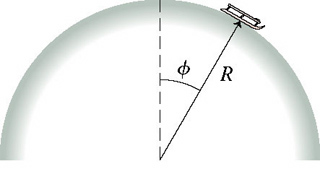# Problem: A sled starts from rest at the top of the frictionless, hemispherical, snow-covered hill shown in the figure. A. Find an expression for the sleds speed when it is at angle ϕexttip{phi }{phi}.B. Use Newtons laws to find the maximum speed the sled can have at angle exttip{phi }{phi} without leaving the surface.C. At what angle exttip{phi _{ m max}}{phi_max} does the sled "fly off" the hill?

###### FREE Expert Solution

In this problem, we'll consider the equation for the conservation of energy:

$\overline{){{\mathbf{K}}}_{{\mathbf{i}}}{\mathbf{+}}{{\mathbf{U}}}_{{\mathbf{i}}}{\mathbf{=}}{{\mathbf{K}}}_{{\mathbf{f}}}{\mathbf{+}}{{\mathbf{U}}}_{{\mathbf{f}}}}$, where K is the kinetic energy and U is the potential energy.

Centripetal force:

$\overline{){{\mathbf{F}}}_{{\mathbf{c}}}{\mathbf{=}}\frac{\mathbf{m}{\mathbf{v}}^{\mathbf{2}}}{\mathbf{r}}}$

We can visualize our problem in the figure:We're told that the sled starts from rest at the top (A) then slides down on the hemisphere.

Let's take the sled to be located at an angle, ϕ at an instant as shown in the figure above.

A is the initial position of the sled and B is the sled's position at angle ϕ.

Due to the potential energy at point A, the sled will acquire kinetic energy.

From the law of conservation of energy:

KA + UA = KB + UB

90% (166 ratings)###### Problem Details

A sled starts from rest at the top of the frictionless, hemispherical, snow-covered hill shown in the figure.A. Find an expression for the sleds speed when it is at angle ϕ.

B. Use Newtons laws to find the maximum speed the sled can have at angle without leaving the surface.

C. At what angle does the sled "fly off" the hill?

Frequently Asked Questions

What scientific concept do you need to know in order to solve this problem?

Our tutors have indicated that to solve this problem you will need to apply the Motion Along Curved Paths concept. You can view video lessons to learn Motion Along Curved Paths. Or if you need more Motion Along Curved Paths practice, you can also practice Motion Along Curved Paths practice problems.

What professor is this problem relevant for?

Based on our data, we think this problem is relevant for Professor O'Donnell's class at VT.